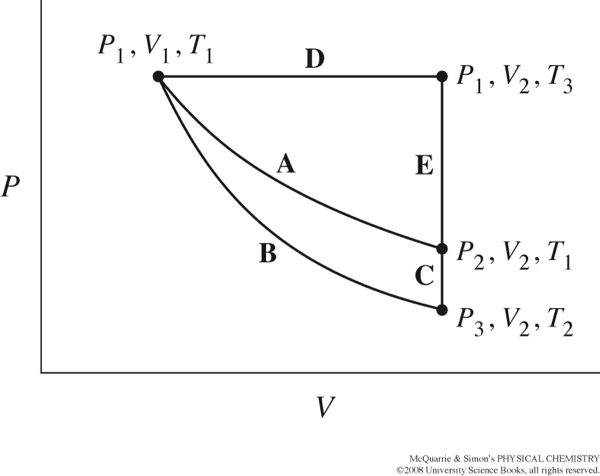# Groupwork 4 Path vs. state functions

Name: ______________________________

Section: _____________________________

Student ID#:__________________________

Work in groups on these problems. You should try to answer the questions without referring to your textbook. If you get stuck, try asking another group for help.

In thermodynamics, some functions we encounter depend on the path followed, and others only on the current state in which we find the system. This groupwork intends to help you gain understanding and appreciation of these concepts.

The figure below from your text, shows several different paths that could be taken as thermodynamic variables, P, T, and V, change.Can you use the same The internal energy, U, is a state function. What is the total change in U when changing from P1, V1, T1 to P1, V2, T3 along path D, followed by the change from P1, V2, T3 to P2, V2, T1 along path E, and then back again along path A to P1, V1, T1? That is, what is ΔUtotal= ΔUD + ΔUE + ΔUA? How did figure this out?

How can you use the value ΔUtotal from the full cycle to find the change in internal energy, ΔU along one part of the cycle, for example ΔUE?

concept if you want to find the total work done, w, or heat evolved, q, along one segment of the cycle? Why or why not?

If you follow path E from P1, V2, T3 to P2, V2, T1, how much work is done by or on the system? (Think about the changes in pressure, volume and temperature and our definition of work). Does this tell you anything about the work done by or on the system for other parts of the cycle?

Consider the cycle from P1, V1, T1 to P1, V2, T3 along path D, followed by the change from P1, V2, T3 to P3, V2, T2 along paths E+C, and then back again along path B to P1, V1, T1?

The change in internal energy along path B is zero, ΔUB=0. How does this simplify your calculation of ΔUtotal = ΔUD + ΔUE + ΔUC + ΔUB for the entire cycle?

Given that ΔU=w+q and ΔUB=0, what must be true for heat evolved/absorbed, and work done on or by the system along path B?

Indicate whether the variable increases (+), decreases (-) or stays the same (0) for each path.

 Path Pressure,$$P$$ Volume,$$V$$ Temperature,$$T$$ Internal Energy $$\Delta U$$ Heat,$$q$$ Work,$$w$$ D E A B C